# Find duplicate rows in a Dataframe based on all or selected columns

• Last Updated : 16 Feb, 2022

In this article, we will be discussing how to find duplicate rows in a Dataframe based on all or a list of columns. For this, we will use Dataframe.duplicated() method of Pandas.

Syntax : DataFrame.duplicated(subset = None, keep = ‘first’)
Parameters:
subset: This Takes a column or list of column label. It’s default value is None. After passing columns, it will consider them only for duplicates.
keep: This Controls how to consider duplicate value. It has only three distinct value and default is ‘first’.

• If ‘first’, This considers first value as unique and rest of the same values as duplicate.
• If ‘last’, This considers last value as unique and rest of the same values as duplicate.
• If ‘False’, This considers all of the same values as duplicates.

Returns: Boolean Series denoting duplicate rows.

Let’s create a simple dataframe with a dictionary of lists, say column names are: ‘Name’, ‘Age’ and ‘City’.

## Python3

 `# Import pandas library``import` `pandas as pd` `# List of Tuples``employees ``=` `[(``'Stuti'``, ``28``, ``'Varanasi'``),``            ``(``'Saumya'``, ``32``, ``'Delhi'``),``            ``(``'Aaditya'``, ``25``, ``'Mumbai'``),``            ``(``'Saumya'``, ``32``, ``'Delhi'``),``            ``(``'Saumya'``, ``32``, ``'Delhi'``),``            ``(``'Saumya'``, ``32``, ``'Mumbai'``),``            ``(``'Aaditya'``, ``40``, ``'Dehradun'``),``            ``(``'Seema'``, ``32``, ``'Delhi'``)``            ``]` `# Creating a DataFrame object``df ``=` `pd.DataFrame(employees,``                  ``columns ``=` `[``'Name'``, ``'Age'``, ``'City'``])` `# Print the Dataframe``df`

Output :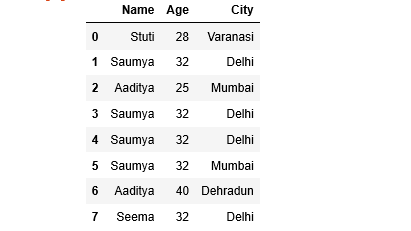Example 1: Select duplicate rows based on all columns.
Here, We do not pass any argument, therefore, it takes default values for both the arguments i.e. subset = None and keep = ‘first’.

## Python3

 `# Import pandas library``import` `pandas as pd` `# List of Tuples``employees ``=` `[(``'Stuti'``, ``28``, ``'Varanasi'``),``            ``(``'Saumya'``, ``32``, ``'Delhi'``),``            ``(``'Aaditya'``, ``25``, ``'Mumbai'``),``            ``(``'Saumya'``, ``32``, ``'Delhi'``),``            ``(``'Saumya'``, ``32``, ``'Delhi'``),``            ``(``'Saumya'``, ``32``, ``'Mumbai'``),``            ``(``'Aaditya'``, ``40``, ``'Dehradun'``),``            ``(``'Seema'``, ``32``, ``'Delhi'``)``            ``]` `# Creating a DataFrame object``df ``=` `pd.DataFrame(employees,``                  ``columns ``=` `[``'Name'``, ``'Age'``, ``'City'``])` `# Selecting duplicate rows except first``# occurrence based on all columns``duplicate ``=` `df[df.duplicated()]` `print``("Duplicate Rows :")` `# Print the resultant Dataframe``duplicate`

Output :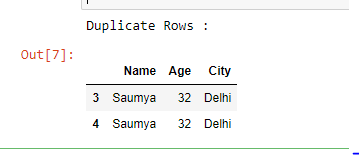Example 2: Select duplicate rows based on all columns.
If you want to consider all duplicates except the last one then pass keep = ‘last’ as an argument.

## Python3

 `# Import pandas library``import` `pandas as pd` `# List of Tuples``employees ``=` `[(``'Stuti'``, ``28``, ``'Varanasi'``),``            ``(``'Saumya'``, ``32``, ``'Delhi'``),``            ``(``'Aaditya'``, ``25``, ``'Mumbai'``),``            ``(``'Saumya'``, ``32``, ``'Delhi'``),``            ``(``'Saumya'``, ``32``, ``'Delhi'``),``            ``(``'Saumya'``, ``32``, ``'Mumbai'``),``            ``(``'Aaditya'``, ``40``, ``'Dehradun'``),``            ``(``'Seema'``, ``32``, ``'Delhi'``)``            ``]` `# Creating a DataFrame object``df ``=` `pd.DataFrame(employees,``                  ``columns ``=` `[``'Name'``, ``'Age'``, ``'City'``])` `# Selecting duplicate rows except last``# occurrence based on all columns.``duplicate ``=` `df[df.duplicated(keep ``=` `'last'``)]` `print``("Duplicate Rows :")` `# Print the resultant Dataframe``duplicate`

Output :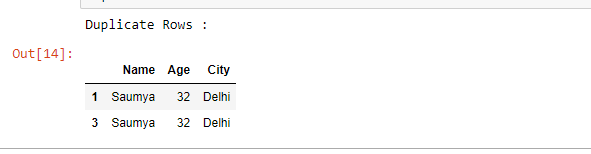Example 3: If you want to select duplicate rows based only on some selected columns then pass the list of column names in subset as an argument.

## Python3

 `# import pandas library``import` `pandas as pd` `# List of Tuples``employees ``=` `[(``'Stuti'``, ``28``, ``'Varanasi'``),``            ``(``'Saumya'``, ``32``, ``'Delhi'``),``            ``(``'Aaditya'``, ``25``, ``'Mumbai'``),``            ``(``'Saumya'``, ``32``, ``'Delhi'``),``            ``(``'Saumya'``, ``32``, ``'Delhi'``),``            ``(``'Saumya'``, ``32``, ``'Mumbai'``),``            ``(``'Aaditya'``, ``40``, ``'Dehradun'``),``            ``(``'Seema'``, ``32``, ``'Delhi'``)``            ``]` `# Creating a DataFrame object``df ``=` `pd.DataFrame(employees,``                  ``columns ``=` `[``'Name'``, ``'Age'``, ``'City'``])` `# Selecting duplicate rows based``# on 'City' column``duplicate ``=` `df[df.duplicated(``'City'``)]` `print``("Duplicate Rows based on City :")` `# Print the resultant Dataframe``duplicate`

Output :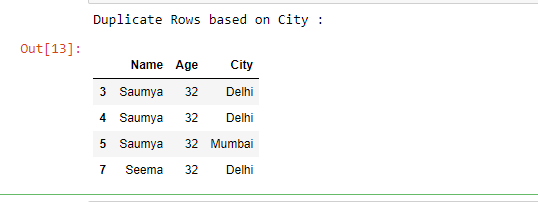Example 4: Select duplicate rows based on more than one column name.

## Python3

 `# import pandas library``import` `pandas as pd` `# List of Tuples``employees ``=` `[(``'Stuti'``, ``28``, ``'Varanasi'``),``            ``(``'Saumya'``, ``32``, ``'Delhi'``),``            ``(``'Aaditya'``, ``25``, ``'Mumbai'``),``            ``(``'Saumya'``, ``32``, ``'Delhi'``),``            ``(``'Saumya'``, ``32``, ``'Delhi'``),``            ``(``'Saumya'``, ``32``, ``'Mumbai'``),``            ``(``'Aaditya'``, ``40``, ``'Dehradun'``),``            ``(``'Seema'``, ``32``, ``'Delhi'``)``            ``]` `# Creating a DataFrame object ``df ``=` `pd.DataFrame(employees,``                   ``columns ``=` `[``'Name'``, ``'Age'``, ``'City'``])` `# Selecting duplicate rows based``# on list of column names``duplicate ``=` `df[df.duplicated([``'Name'``, ``'Age'``])]` `print``("Duplicate Rows based on Name ``and` `Age :")` `# Print the resultant Dataframe``duplicate`

Output :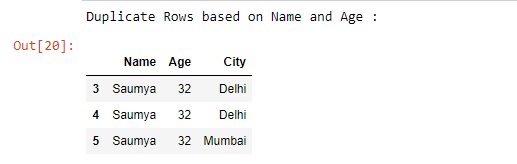My Personal Notes arrow_drop_up